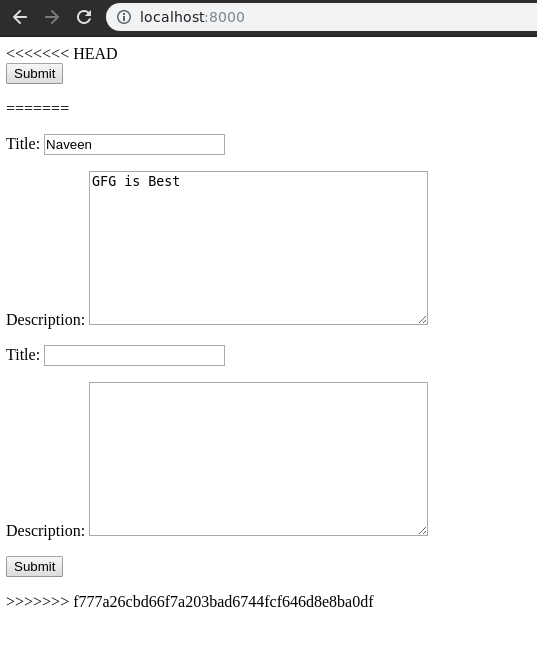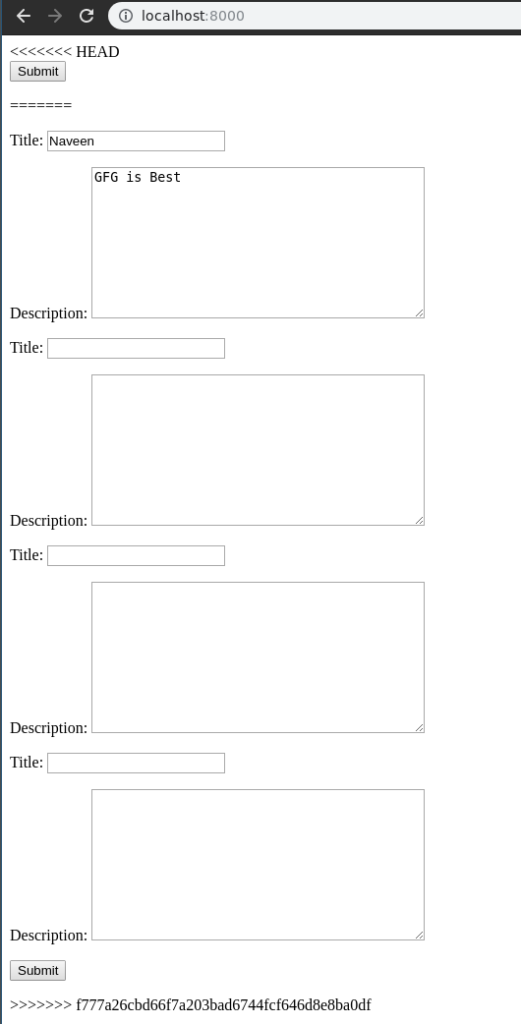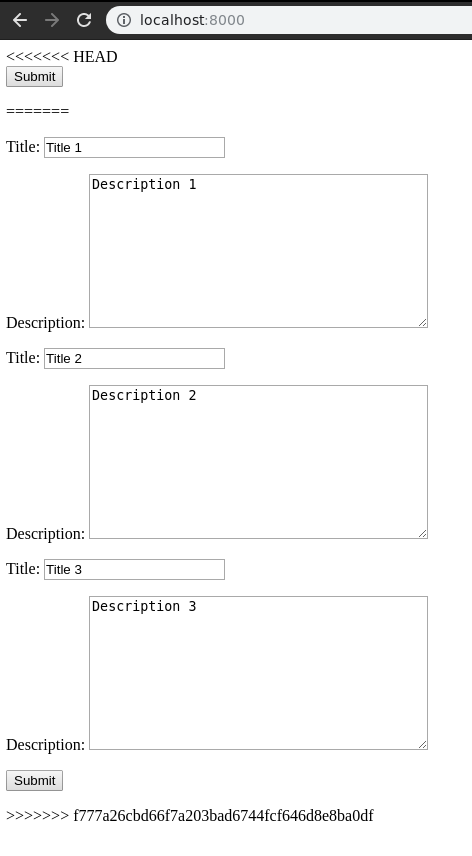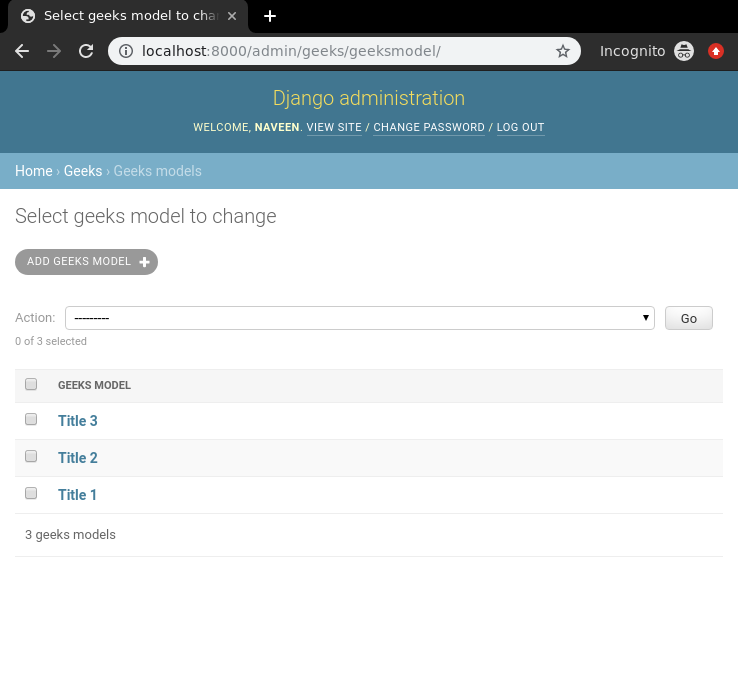# Django ModelFormSets

ModelFormsets in a Django is an advanced way of handling multiple forms created using a model and use them to create model instances. In other words, ModelFormsets are a group of forms in Django. One might want to initialize multiple forms on a single page all of which may involve multiple POST requests, for example

```class GeeksModel(models.Model):
title = models.CharField(max_length = 200)
description = models.TextField()
```

Now if one wants to create a modelformset for this model, modelformset_factory needs to be used. A formset is a layer of abstraction to work with multiple forms on the same page. It can be best compared to a data grid.

```from django.forms import formset_factory
GeeksFormSet = modelformset_factory(GeeksModel)
```

### Creating and using Django ModelFormsets

Illustration of Rendering Django ModelFormsets manually using an Example. Consider a project named `geeksforgeeks` having an app named `geeks`.

Refer to the following articles to check how to create a project and an app in Django.

In your geeks app make a new file called models.py where you would be making all your models. To create a Django model you need to use Django Models. Let’s demonstrate how,
In your` models.py` Enter the following,

 `# import the standard Django Model ` `# from built-in library ` `from` `django.db ``import` `models ` `  `  `# declare a new model with a name "GeeksModel" ` `class` `GeeksModel(models.Model): ` ` `  `    ``# fields of the model ` `    ``title ``=` `models.CharField(max_length ``=` `200``) ` `    ``description ``=` `models.TextField() ` ` `  `    ``# renames the instances of the model ` `    ``# with their title name ` `    ``def` `__str__(``self``): ` `        ``return` `self``.title `

Let’s explain what exactly is happening, left side denotes the name of the field and to right of it, you define various functionalities of an input field correspondingly. A field’s syntax is denoted as
Syntax :

`Field_name = models.FieldType(attributes)`

Now to create a simple formset of this form, move to views.py and create a `formset_view` as below.

 `from` `django.shortcuts ``import` `render ` ` `  `# relative import of forms ` `from` `.forms ``import` `GeeksForm ` ` `  `# importing formset_factory ` `from` `django.forms ``import` `formset_factory ` ` `  `def` `formset_view(request): ` `    ``context ``=``{} ` ` `  `    ``# creating a formset ` `    ``GeeksFormSet ``=` `modelformset_factory(GeeksForm) ` `    ``formset ``=` `GeeksFormSet() ` `     `  `    ``# Add the formset to context dictionary ` `    ``context[``'formset'``]``=` `formset ` `    ``return` `render(request, ``"home.html"``, context) `

To render the formset through HTML, create a html file “home.html”. Now let’s edit `templates > home.html`

 `<``form` `method``=``"POST"` `enctype``=``"multipart/form-data"``> ` `    ``{% csrf_token %} ` `    ``{{ formset.as_p }} ` `    ``<``input` `type``=``"submit"` `value``=``"Submit"``> ` ` `

All set to check if our formset is working or not let’s visit http://localhost:8000/.Our `modelformset` is working completely. Let’s learn how to modify this formset to use extra features of this formset.

### How to create Multiple forms using Django ModelFormsets

Django formsets are used to handle multiple instances of a form. One can create multiple forms easily using `extra` attribute of Django Formsets. In geeks/views.py,

 `from` `django.shortcuts ``import` `render ` ` `  `# relative import of forms ` `from` `.models ``import` `GeeksModel ` ` `  `# importing formset_factory ` `from` `django.forms ``import` `modelformset_factory ` ` `  `def` `modelformset_view(request): ` `    ``context ``=``{} ` ` `  `    ``# creating a formset and 5 instances of GeeksForm ` `    ``GeeksFormSet ``=` `modelformset_factory(GeeksModel, fields ``=``[``'title'``, ``'description'``], extra ``=` `3``) ` `    ``formset ``=` `GeeksFormSet() ` ` `  `             `  `    ``# Add the formset to context dictionary ` `    ``context[``'formset'``]``=` `formset ` `    ``return` `render(request, ``"home.html"``, context) `

The keyword argument `extra` makes multiple copies of same form. If one wants to create 5 forms enter `extra = 5` and similarly for others. Visit http://localhost:8000/ to check if 5 forms are created.### Handling Multiple forms using Django Formsets

Creating a form is much easier than handling the data entered into those fields at the back end. Let’s try to demonstrate how one can easily use the data of a model formset in a view. When trying to handle formset, Django formsets required one extra argument {{ formset.management_data }}. To know more about Management data, Understanding the ManagementForm.
In `templates/home.html`,

 `<``form` `method``=``"POST"` `enctype``=``"multipart/form-data"``> ` `     `  `    ``` `    ``{{ formset.management_data }} ` ` `  `    ``` `    ``{% csrf_token %} ` ` `  `    ``` `    ``{{ formset.as_p }} ` `     `  `    ``<``input` `type``=``"submit"` `value``=``"Submit"``> ` ` `

Now to check how and what type of data is being rendered edit formset_view to print the data. In `geeks/view.py`,

 `from` `django.shortcuts ``import` `render ` ` `  `# relative import of forms ` `from` `.forms ``import` `GeeksForm ` ` `  `# importing formset_factory ` `from` `django.forms ``import` `formset_factory ` ` `  `def` `formset_view(request): ` `    ``context ``=``{} ` ` `  `    ``# creating a formset and 5 instances of GeeksForm ` `    ``GeeksFormSet ``=` `formset_factory(GeeksForm, extra ``=` `3``) ` `    ``formset ``=` `GeeksFormSet(request.POST ``or` `None``) ` `     `  `    ``# print formset data if it is valid ` `    ``if` `formset.is_valid(): ` `        ``for` `form ``in` `formset: ` `            ``print``(form.cleaned_data) ` `             `  `    ``# Add the formset to context dictionary ` `    ``context[``'formset'``]``=` `formset ` `    ``return` `render(request, ``"home.html"``, context) `

Now let’s try to enter data in the formset through http://localhost:8000/Hit submit and data will be saved in GeeksModel where server is running. One can use this data in any manner conveniently now.My Personal Notes arrow_drop_upCheck out this Author's contributed articles.

If you like GeeksforGeeks and would like to contribute, you can also write an article using contribute.geeksforgeeks.org or mail your article to contribute@geeksforgeeks.org. See your article appearing on the GeeksforGeeks main page and help other Geeks.

Please Improve this article if you find anything incorrect by clicking on the "Improve Article" button below.

Article Tags :

Be the First to upvote.

Please write to us at contribute@geeksforgeeks.org to report any issue with the above content.